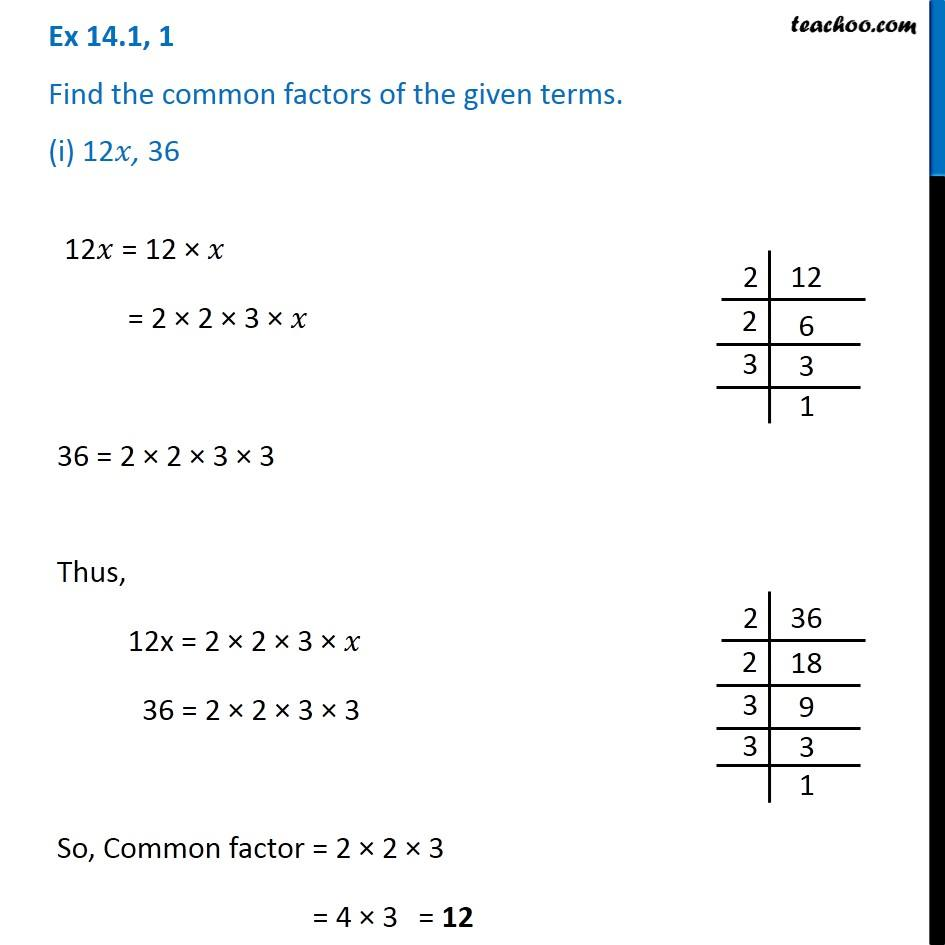Ex 14.1

Chapter 14 Class 8 Factorisation
Serial order wise641 students have Teachoo Black. What are you waiting for?

### Transcript

Ex 14.1, 1 Find the common factors of the given terms. (i) 12𝑥, 36 12𝑥 = 12 × 𝑥 = 2 × 2 × 3 × 𝑥 36 = 2 × 2 × 3 × 3 Thus, 12x = 2 × 2 × 3 × 𝑥 36 = 2 × 2 × 3 × 3 So, Common factor = 2 × 2 × 3 = 4 × 3 = 12